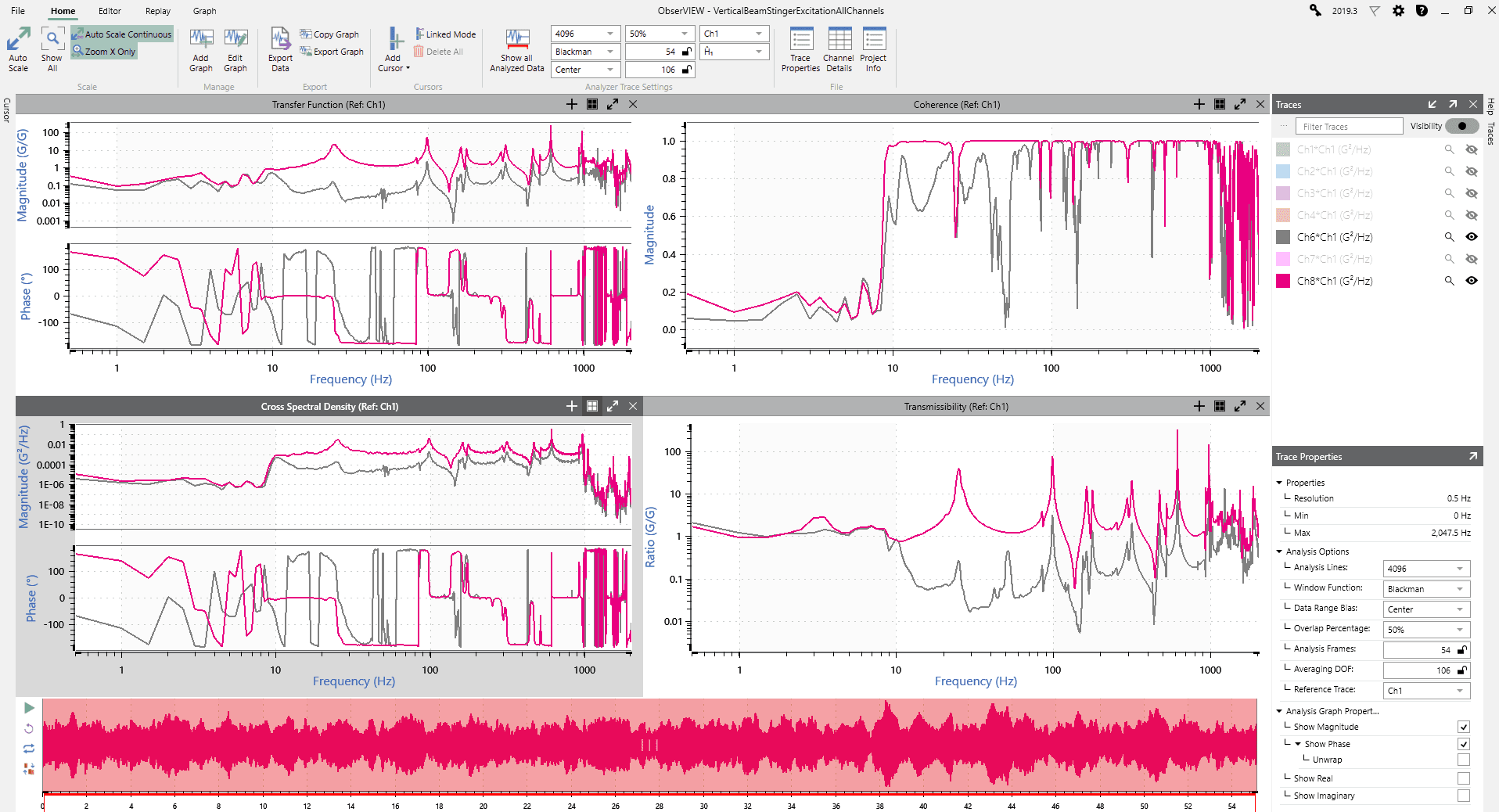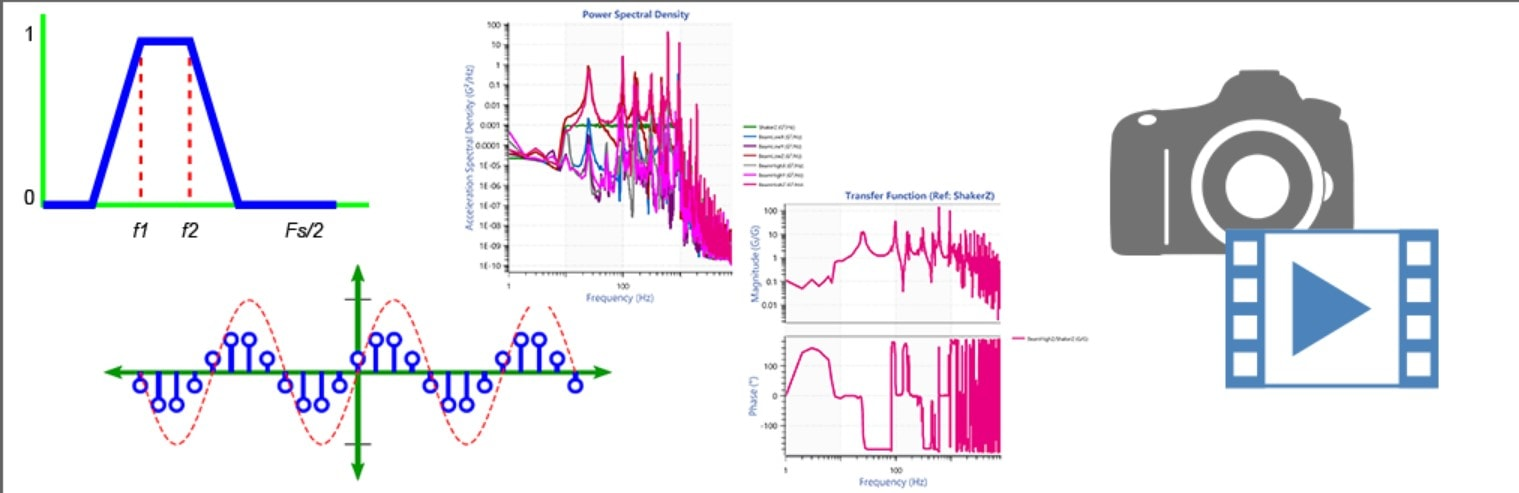Take advantage of Resampling, Filtering, Advanced Data Analysis (PSD, Transfer Function, etc.), and Multimedia Sync. A free trial of an Advanced License can be obtained by clicking Request Trial in ObserVIEW.

Further graphing capabilities include Coherence, Transfer Function, Cross Spectral Density, and Transmissibility### PSD Analysis

Power refers to the magnitude of the PSD is the mean-square value of the signal being analyzed. Spectral refers to a PSD being a function of frequency. Density refers to the power in a band of frequencies under the PSD curve in that band.

### PSD Defined

In the term Power Spectral Density, each word is chosen to represent an essential component of the PSD.

Power refers to the fact that the magnitude of the PSD is the mean-square value of the signal being analyzed. It does not refer to the physical quantity power (as in watts or horsepower). But since power is proportional to the mean-square value of some quantity (such as the square of current or voltage in an electrical circuit), the mean-square value of any quantity has become known as the power of that quantity.

Spectral refers to the fact that the PSD is a function of frequency. The PSD represents the distribution of a signal over a spectrum of frequencies just like a rainbow represents the distribution of light over a spectrum of wavelengths (or colors).

Density refers to the fact that power in a band of frequencies (from a from frequency f1 to a frequency f2) is the area under the PSD curve in that band.

Since the name PSD does not include the quantity being measured, the word power is sometimes replaced by the name of the quantity being measured. For example, the PSD of an acceleration signal is sometimes referred to as the Acceleration Spectral Density.

### Auto Spectral Density (ASD) / Power Spectral Density (PSD)

The PSD plot shows how much power is in a given band of frequencies. In particular, the area under the PSD curve from frequency f1 to frequency f2 is the power (RMS) in that band of frequencies.

The PSD can be used to find resonant frequencies located in a random signal.

### The Statistical PSD Analysis View

PSD shows how correlated (“related”, “statistically connected”, “influenced” by other parts of) a signal is in relation to itself. A signal with “flat” PSD indicates that it is very uncorrelated (“unrelated”, “statistically unconnected”, are not “influenced” by each other, … like consecutive tosses of a fair die). Whereas a signal with some kind of “main lobe” has some degree of correlation. Generally speaking,

• the wider this main lobe is, the more uncorrelated the signal is; and
• the narrower the main lobe is, the more correlated.### Transfer Function

H(f) may be used to find a resonance or an antiresonance in a system. That is, it shows around what frequencies (if any) there is a sudden increase (resonance) or decrease (anti-resonance) in the spectral power through a device or system. A transfer function estimate Ĥ(f) is an estimate of the true transfer function H(f), which in general is not known (but can be estimated). Averaging more analysis frames together converges the estimate to the actual transfer function in an appropriate model (LTI, wide sense stationary).

### Coherence

Is an indication of how closely a pair of signals x and y are statistically related. That is, it is an indication of how closely x “coheres” or “sticks to” y—how much influence events at x and events at y have on each other. The magnitude of coherence will always be between 0 (no influence) and 1 (direct influence). Use coherence to measure the validity of the transfer function at each frequency.

### Cross Spectral Density

Shows how much power is in a given band in the frequency domain of a pair of signals that have been multiplied in the frequency domain. In particular, the area under the CSD curve from frequency f1 to frequency f2 is the power (RMS) in that band of frequencies.

### Transmissibility

Similar to transfer function estimates, Transmissibility may be used to find a resonance or an antiresonance in a system. That is, it shows around what frequencies (if any) there is a sudden increase (resonance) or decrease (antiresonance) in the spectral power through a device or system (Prefer Transfer Function).